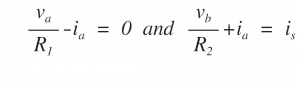Home > dccircuits > nodal analysis

# Nodal analysis

In the nodal analysis, Kirchhoff's current law is used to write the equilibrium equations. A node is defined as a junction of two or more branches. If we define one node of the network as a reference node (a point of zero potential or ground), the remaining nodes of the network will have a fixed potential relative to this reference. Equations relating to all nodes except for the reference node can be written by applying KCL. Refering to the circuit shown in Figure below, we can arbitrarily choose any node as the reference node. However, it is convenient to choose the node with most connected branches. Hence, node 3 is chosen as the reference node here.It is seen from the network of Figure that there are three nodes.

Hence, number of equations based on KCL will be total number of nodes minus one.That is, in the present context, we will have only two KCL equations referred to as node equations. For applying KCL at node 1 and node 2, we assume that all the currents leave these nodes as shown in below Figures.

Applying KCL at node 1 and 2, we find that

Steps for nodal analysis :

1. Identify and mark all the nodes (including the reference node) and the corresponding node voltages.
2. Mark all the branch currents.
3. Write the KCL equation at every node in terms of conductance (G), node voltages and currents.
4. Transform the KCL equations into the matrix form and solve them using the Cramer's rule to obtain various node voltages.

Supernode

In order to understand the concept of a supernode, let us consider an electrical circuit as shown in Fig.

Applying KVL clockwise to the loop containing R1, voltage source and R2,

To account for the fact that the source voltage is known, we consider both a and b as part of one larger node represented by the dotted ellipse as shown in Figure.

We need a larger node because a and b are dependent. This larger node is called the supernode.

Applying KCL at nodes a and b,

we get,Adding the above two equations, we find that,

When we apply KCL at the supernode, mentally imagine that the voltage source is removed from the the circuit of Figure but the voltage at nodes a and b are held at and respectively. In other words, by applying KCL at supernode, we obtain

The equation is the same equation. As in supermesh, the KCL for supernode eliminates the problem of dealing with a current through a voltage source.

Procedure for using supernode:

1. Use it when a branch between non-reference nodes is connected by an independent or a dependent voltage source.
2. Enclose the voltage source and the two connecting nodes inside a dotted ellipse to form the supernode.
3. Write the constraint equation that defines the voltage relationship between the two non-reference node as a result of the presence of the voltage source.
4. Write the KCL equation at the supernode.
5. If the voltage source is dependent, then the constraint equation for the dependent source is also needed.